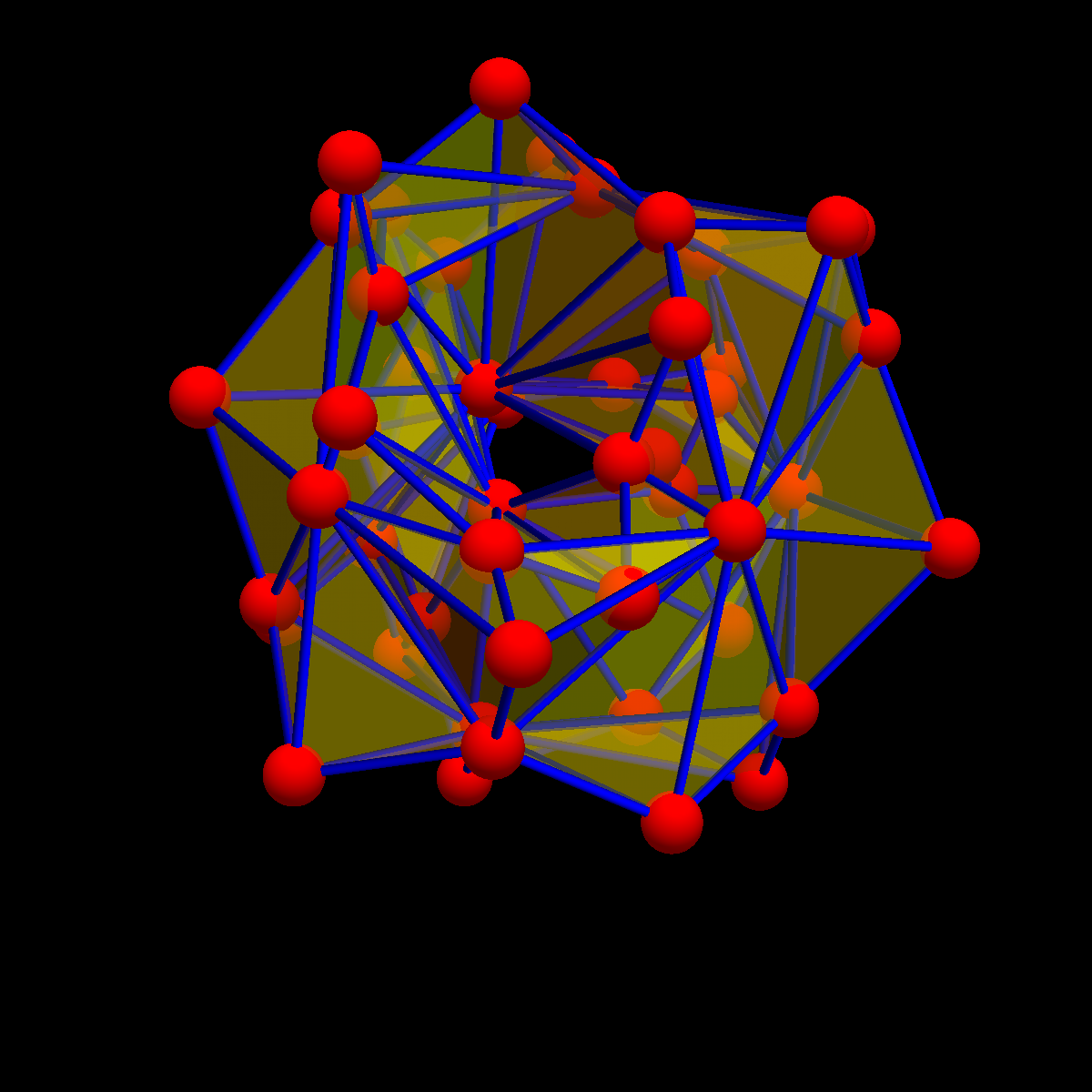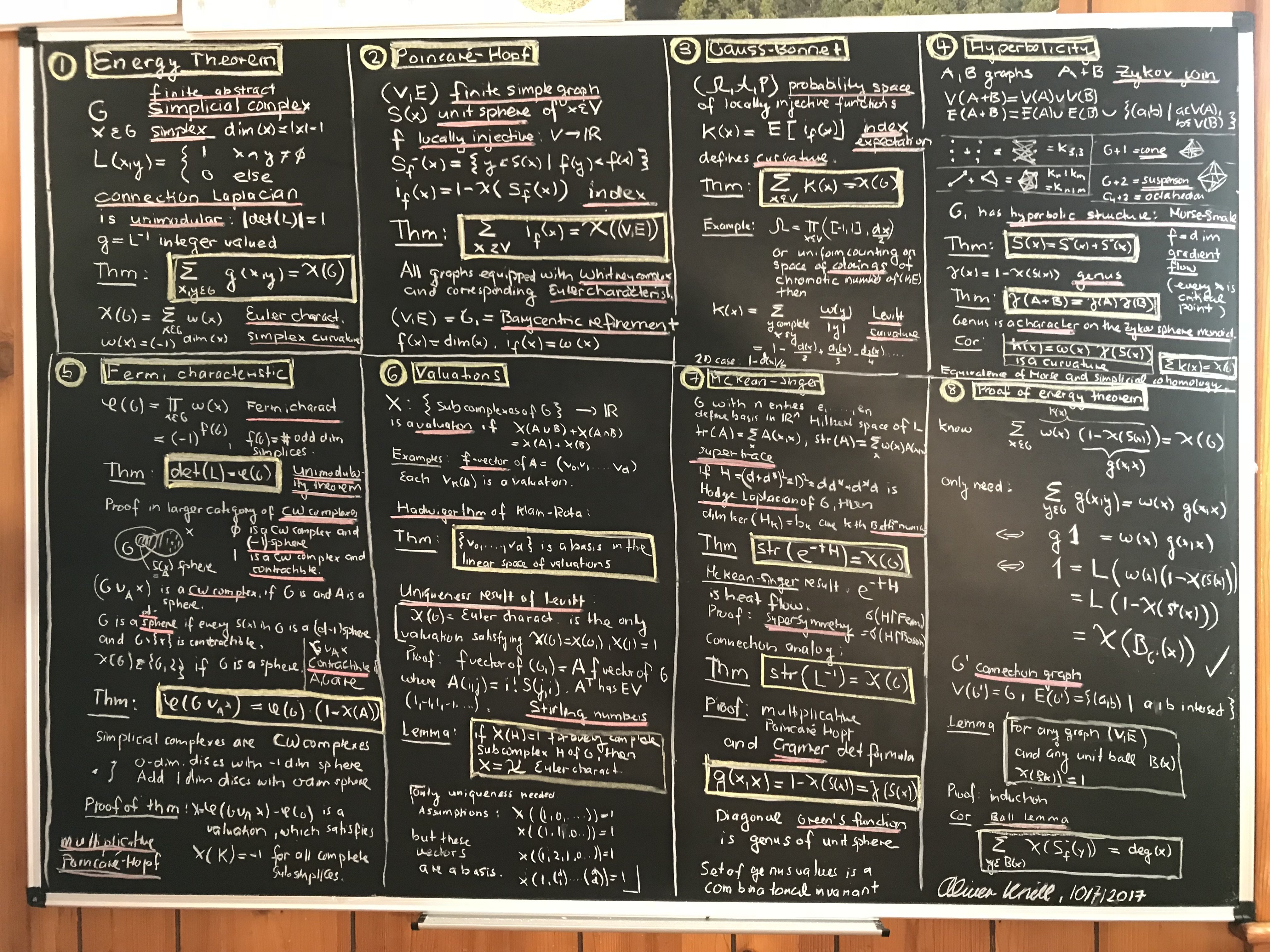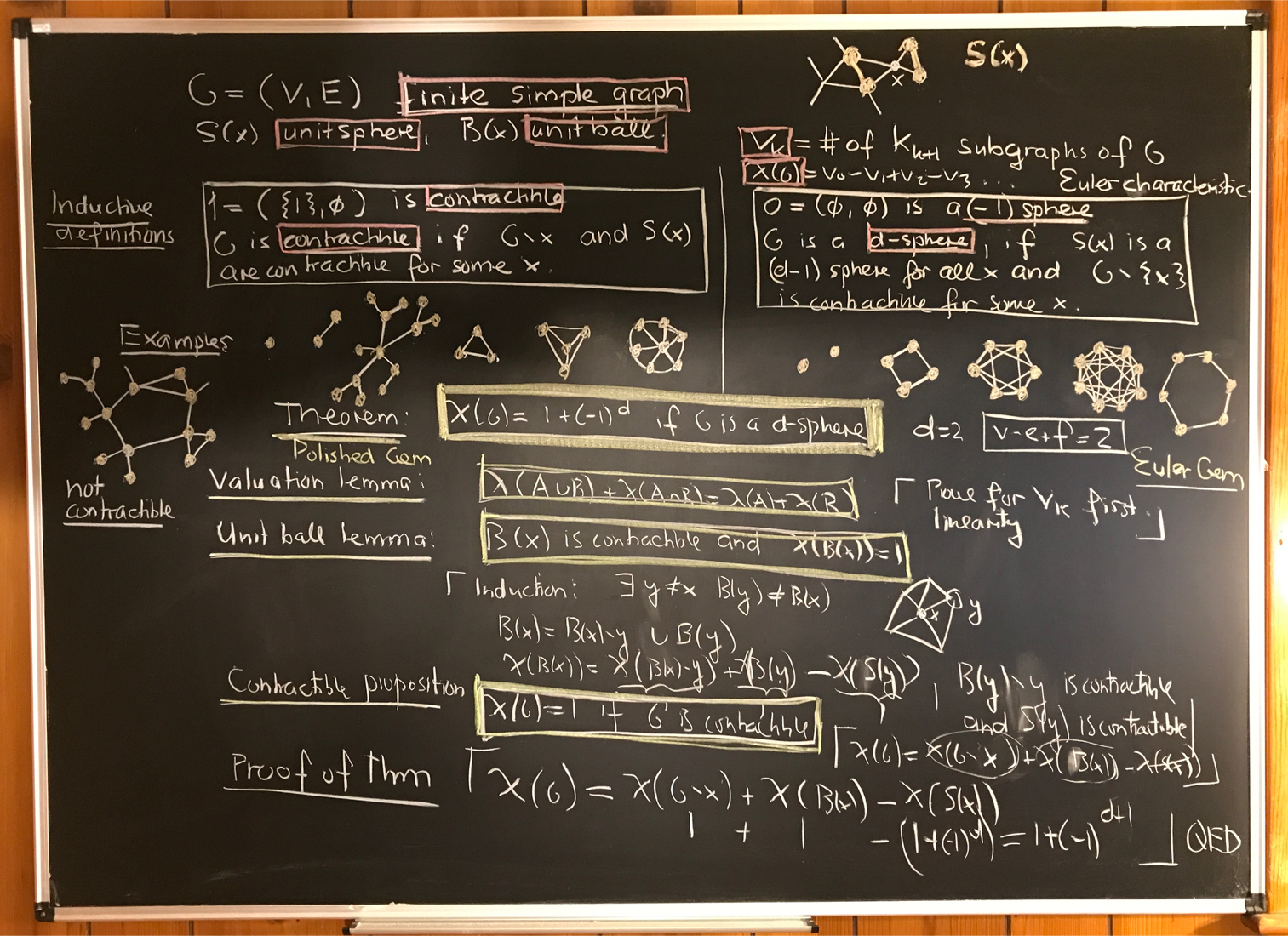# The energy of a simplicial complex

• November 19, 2020 : A blog entry in the Wolfram community page. Mathematica Notebook .nb.
• October 19, 2020 ArXiv: Green Functions of Energized Complexes. Here is some code illustrating the four theorems:
``` G={{1},{2},{3},{4},{1,2},{2,3},{3,4}}; n=Length[G]; e = {x,y,z,a,b,c,d};
L0=Table[If[Equal[Intersection[G[[k]],G[[l]]],{}],0,1],{k,n},{l,n}];
energy[A_]:=If[A=={},0,Sum[e[[Position[G,A[[k]]][[1,1]]]],{k,Length[A]}]];
S=Table[-(-1)^Length[G[[k]]]*If[k ==l,1,0],{k,n},{l,n}];  w[k_]:=S[[k,k]];
star[x_]:=Module[{u={}},Do[v=G[[k]];If[SubsetQ[v,x],u=Append[u,v]],{k,n}];u];
core[x_]:=Module[{u={}},Do[v=G[[k]];If[SubsetQ[x,v],u=Append[u,v]],{k,n}];u];
Wminus    = Table[Intersection[core[G[[k]]],core[G[[l]]]],{k,n},{l,n}];
Wplus     = Table[Intersection[star[G[[k]]],star[G[[l]]]],{k,n},{l,n}];
Lminus    = Table[energy[Wminus[[k,l]]],    {k,n},{l,n}];  L  =      Lminus;
Lplus     = Table[energy[Wplus[[k,l]]],     {k,n},{l,n}];  g  =   S.Lplus.S;
Wu        = Sum[e[[k]] e[[l]] L0[[k,l]],{k,n},{l,n}];
Mo        = Sum[g[[k,l]]^2 w[k] w[l] ,{k,n},{l,n}];
Print["Theorem 1: ",Simplify[energy[G]-Sum[g[[k,l]],{k,n},{l,n}]]];
Print["Theorem 2: ",Simplify[Wu  - Mo]];
Print["Theorem 3: ",Product[e[[k]],{k,n}] - Det[g]];
Print["Theorem 4: ",MatrixForm[Simplify[g.L]]];
```
Here is the output:
```Theorem 1: 0
Theorem 2: 0
Theorem 3: 0
Theorem 4:  2                                            2    2
x          0          0          0          -b  + x    0          0

2                                 2    2     2    2
0          y          0          0          -b  + y    -c  + y    0

2                                 2    2     2    2
0          0          z          0          0          -c  + z    -d  + z

2                                2    2
0          0          0          a          0          0          a  - d

2
0          0          0          0          b          0          0

2
0          0          0          0          0          c          0

2
0          0          0          0          0          0          d

```
• August 24, 2020: The sweet simplicial complex article contains some code. (Sweet by the way because C6H12O6 matches with division algebras coming up there). The following code computes the permutation group generated by geometric phase changes. If we turn the field value at a simplex by 360 degrees this produces a permutation of the eigenvalues. All these permutations generate a group.
```Generate[A_]:=Delete[Union[Sort[Flatten[Map[Subsets,A],1]]],1];
G = Generate[{{1,2},{2,3},{3,4},{5,6}}]; n=Length[G];  Q=SubsetQ;
S=Table[-(-1)^Length[G[[k]]]*If[k ==l,1,0],{k,n},{l,n}];
star[x_]:=Module[{u={}},Do[v=G[[k]];If[Q[v,x],u=Append[u,v]],{k,n}];u];
core[x_]:=Module[{u={}},Do[v=G[[k]];If[Q[x,v],u=Append[u,v]],{k,n}];u];
Wminus    = Table[Intersection[core[G[[k]]],core[G[[l]]]],{k,n},{l,n}];
Wplus     = Table[Intersection[star[G[[k]]],star[G[[l]]]],{k,n},{l,n}];
e=Table[Exp[1.0*2Pi I k/n],{k,n}];
EN[A_]:=If[A=={},0,Sum[e[[Position[G,A[[k]]][[1,1]]]],{k,Length[A]}]];
L=  Table[EN[Wminus[[k,l]]],   {k,n},{l,n}];   V=Eigenvalues[1.0*L];
g=S.Table[EN[Wplus[[k,l]]],    {k,n},{l,n}].S; Chop[Conjugate[g].L];
TrackEigenvalue[m_,w_]:=Module[{t=0,XX=V[[m]],q=1},
Do[ e1=e;  e1[[w]]=e[[w]]*Exp[I t];
EN[A_]:=If[A=={},0,Sum[e1[[Position[G,A[[k]]][[1,1]]]],{k,Length[A]}]];
L1=Table[EN[Wminus[[k,l]]], {k,n},{l,n}]; V1=Eigenvalues[1.0*L1];
min=n; Do[If[Less[Abs[XX-V1[[k]]],min],min=Abs[XX-V1[[k]]];q=k],{k,n}];
XX=V1[[q]], {t,0,2Pi,2Pi/500}];
min=n;Do[If[Less[Abs[XX-V[[k]]],min],min=Abs[XX-V[[k]]];q=k],{k,n}];q];
Perm[w_]:=Table[TrackEigenvalue[m,w],{m,n}];
GroupOrder[PermutationGroup[Table[PermutationCycles[Perm[w]],{w,n}]]]
```
This is also related to flat Kähler manifolds. The map assigning to the wave the eigenvalues of the operator L is a linear paramerization r of a complex n dimensional Kaehler manifold. It is interesting to look at the determinant of the flat Kaehler form g=drT dr. Here is the code:
```Generate[A_]:=Delete[Union[Sort[Flatten[Map[Subsets,A],1]]],1];
G1=Generate[{{1,2,3,4,5},{3,4,5,6,7}}];  G2=Generate[{Range}];
G=G2; n=Length[G]; SQ=SubsetQ;
c[x_]:=Module[{u={}},Do[v=G[[k]];If[SQ[x,v],u=Append[u,v]],{k,n}];u];
Wminus = Table[Intersection[c[G[[k]]],c[G[[l]]]],{k,n},{l,n}];
QQ[e_]:=Module[{en,L},
en[A_]:=If[A=={},0,Sum[e[[Position[G,A[[k]]][[1,1]]]],{k,Length[A]}]];
L = Table[en[Wminus[[k,l]]],  {k,n},{l,n}]; Flatten[L]];
Id=IdentityMatrix[n]; A=Transpose[Table[QQ[Id[[k]]],{k,n}]];
Kaehler=Transpose[A].A;
Print[{Det[Kaehler],"Factors:",FactorInteger[Det[Kaehler]]}];
```
• August, 2020: after a bit of work on classical diff geometry, a bit more work on energy: A geometric phase phenomenon detected. Here is just the code for computing the spectrum in the complex case: Here is the poem as a text file if copy paste should not work
```(* Connection Laplacian defined by a complex wave, Oliver Knill, 1/8/2020  *)
Generate[A_]:=Delete[Union[Sort[Flatten[Map[Subsets,A],1]]],1];
RandomSets[n_,m_]:=Module[{A={},X=Range[n],k},Do[k:=1+Random[Integer,n-1];
A=Append[A,Union[RandomChoice[X,k]]],{m}];Sort[Generate[A]]];
G=RandomSets[5,9]; n=Length[G];
S=Table[-(-1)^Length[G[[k]]]*If[k ==l,1,0],{k,n},{l,n}]; (* Super matrix   *)
star[x_]:=Module[{u={}},Do[v=G[[k]];If[SubsetQ[v,x],u=Append[u,v]],{k,n}];u];
core[x_]:=Module[{u={}},Do[v=G[[k]];If[SubsetQ[x,v],u=Append[u,v]],{k,n}];u];
Wminus     = Table[Intersection[core[G[[k]]],core[G[[l]]]],{k,n},{l,n}];
Wplus      = Table[Intersection[star[G[[k]]],star[G[[l]]]],{k,n},{l,n}];
e=Table[Exp[2Pi I Random[]],{k,n}];
energy[A_]:=If[A=={},0,Sum[e[[Position[G,A[[k]]][[1,1]]]],{k,Length[A]}]];
Lminus     = Table[energy[Wminus[[k,l]]],    {k,n},{l,n}];  L  =      Lminus;
Lplus      = Table[energy[Wplus[[k,l]]],     {k,n},{l,n}];  g  =   S.Lplus.S;
Print[{
Chop[Total[Flatten[g]] - Total[e]],                    (* Energy Thm       *)
Chop[Tr[S.g] -Total[e]],                               (* McKean-Singer Thm*)
Chop[{Det[g],Det[L],N[Product[e[[k]],{k,n}]]}],        (* Unimodularity Thm*)
N[Total[Flatten[Conjugate[g].L -IdentityMatrix[n]]]]}];(* Green-Star Id    *)
```
• April, 2020: The Energy article is up for some time.
• August 19 2019: A generalization in the form of energized simplicial complexes. See also the Blog entry with some code for sets of sets. The energy theorem in general fails for sets of sets and needs a simplicial complex but we have isospectral integer quadratic forms in the more general frame work of finite sets of sets:
```R[n_,m_]:=Module[{A={},X=Range[n],k},Do[k:=1+Random[Integer,n-1];
A=Append[A,Union[RandomChoice[X,k]]],{m}];Sort[Union[A]]];
G=R[6,10];n=Length[G];e=Table[1,{n}];
energy[A_]:=If[A=={},0,Sum[e[[Position[G,A[[k]]][[1,1]]]],{k,Length[A]}]];
star[x_]:=Module[{u={}},Do[v=G[[k]];If[SubsetQ[v,x],u=Append[u,v]],{k,n}];u];
core[x_]:=Module[{u={}},Do[v=G[[k]];If[SubsetQ[x,v],u=Append[u,v]],{k,n}];u];
Wminus=Table[Intersection[core[G[[k]]],core[G[[l]]]],{k,n},{l,n}];
Wplus=Table[Intersection[star[G[[k]]],star[G[[l]]]],{k,n},{l,n}];
Lminus=Table[energy[Wminus[[k,l]]],{k,n},{l,n}];
Lplus=Table[energy[Wplus[[k,l]]],{k,n},{l,n}];
U=Graph[Flatten[Table[Table[G[[k]]<->G[[l]],{Lminus[[k,l]]}],{k,n},{l,k,n}]]];
V=Graph[Flatten[Table[Table[G[[k]]<->G[[l]],{Lplus[[k,l]]}],{k,n},{l,k,n}]]];
Print["Isospectral:",Eigenvalues[Lminus]==Eigenvalues[Lplus]];
```
• July 21, 2019: The counting matrix of a simplicial complex (ArXiv), local [PDF]. About a Bosonic sibling of the connection Laplacian. The matrix is in SL(n,Z) and (very exciting) leads to a zeta function which always satisfies a functional equation. Here is the Mathematica code included:
```Generate[A_]:=Delete[Union[Sort[Flatten[Map[Subsets,A],1]]],1];
R[n_,m_]:=Module[{A={},X=Range[n],k},Do[k:=1+Random[Integer,n-1];
A=Append[A,Union[RandomChoice[X,k]]],{m}];Generate[A]];
G=R[6,9];n=Length[G]; G=Sort[G];                    w[x_]:=-(-1)^Length[x];
star[x_]:=Module[{u={}},Do[v=G[[k]];If[SubsetQ[v,x],u=Append[u,v]],{k,n}];u];
core[x_]:=Module[{u={}},Do[v=G[[k]];If[SubsetQ[x,v],u=Append[u,v]],{k,n}];u];
Wminus=Table[Intersection[core[G[[k]]],core[G[[l]]]],{k,n},{l,n}];
Wplus =Table[Intersection[star[G[[k]]],star[G[[l]]]],{k,n},{l,n}];
K = Table[                    Length[Wminus[[k,l]]],{k,n},{l,n}];
KI= Table[w[G[[k]]]*w[G[[l]]]*Length[Wplus[[k,l]]] ,{k,n},{l,n}];
EV = Sort[Eigenvalues[1.0*K]]; Clear[s]; ZetaFunction=Total[EV^(-s)];
CharPol=CoefficientList[CharacteristicPolynomial[K,s],s];
Print["Green Star formula: ",Simplify[K.KI==IdentityMatrix[n]]];
Print["Energy Theorem:     ",Total[Flatten[KI]]==Length[G]];
Print["Spectral Symmetry:  ",CharPol==(-1)^n*Reverse[CharPol]];
```
• July 7, 2019: The energy of a simplicial complex local copy [PDF] (with updates). This is a new clean write-up containing the unimodularity theorem, energy theorem, hear Euler characteristic theorem and tensor representation theorem. These results were never submitted. This is an attempt for a publication. I feel that it could be an important result which requires to be in a place so that it can be used.

• March 22, 2018: Some new slides:
The energy theorem tells that the sum over all g(x,y) is the Euler characteristic of a complex G, if g is the Green's function, the inverse of the connection matrix of G. Here is a rehearsal of 10/9/2017 for a talk on 10/10/2017. It was proven here and generalized here.
 I) The notation {(12),(23),(1),(2),(3)} saves curly brackets and commas in {{1,2},{2,3},{1},{2},{3}}. II) The fact that omega(x) = i(x) is an index was understated a bit. It verifies in full generality that the Euler characteristic is invariant under Barycentric refinement G -> (V,E)=(G,{(a,b) | a subset b or b subset a}). This follows also from the eigenvector structure of the explicit Barycentric refinement operator involving Stirling numbers. III) Integral geometric approaches to Gauss-Bonnet have tradition: the Blaschke-Chern-Banchoff generation line illustrates this. I asked at various occasions what curvature we get if P is the normalized volume measure on a compact Riemannian manifold and for every x, the heat signature function y -> exp(-t L)(x,h) or the potentials y -> g(x,y) for the Green function is taken. IV) A hyperbolic structure defines a Morse cohomology. Almost by definition, Morse cohomology for the gradient flow of the dimension is simplicial cohomology of G. V) The proof of the multiplicative Poincare-Hopf formula needs the valuation argument that psi(G+x) = 0 if we glue a new cell along a complete complex. This can be analyzed by looking at the cycle structure of det(L) which is the Fredholm determinant det(1+A) of the adjacency matrix: the Fredholm determinant of the adjacency matrix of a complete graph is zero. VI) The talk mentions Boolean algebra. It is only a Boolean lattice. The fact that the class of subcomplexes does not form a Boolean algebra is a stumbling block for proving unimodularity algebraically. VII) In discrete McKean-Singer, str(L^-t))=X(G) only holds for t=1 unlike in the Hodge case, where it is a manifestation of super symmetry. The multiplicative story does not have supersymmetry. Actually, the energy is the discrepancy. VIII) There is more to discrete potential theory: the uniform measure maximizes entropy. One can look at the minimal energy mass on a subcomplex as in classical potential theory. Entropy and Euler characteristic are both natural (uniqueness theorems of Shannon for entropy and Meyer of Euler characteristic). It leads to Helmholtz free energy for which catastrophic phase transitions (blue-sky and pitchfork bifurcations) occur when changing temperature.
 Below is Mathematica code computing the energy and Euler characteristic for a complex generated by a set A. The example given is the Klein bottle, a complex of Euler characteristic 0, Fermi characteristic 1 and f-vector (8,24,16). To the right, is the graph G1 whose Whitney complex is the Barycentric refinement as a complex. We think in the graph. Almost all textbooks of the 20th century treat graphs as 1-dimensional simplicial complexes. There is more to them. Graphs carry many interesting simplicial complex structures. Historically, in the Petersburg bridge problem which was the birth of graph theory, Euler introduced graphs as a model of a 2-dimensional CW complex. The point of view of topological graph theory is to see the 2-dim cells from a graph embedding. For general networks, a natural Whitney clique complex offers itself, rather than the 1-skeleton which treats a graph as a one-dimensional "curve". One can think of a simplicial complex structure on a graph in a similar way as a topological or measure theoretical or order or sheave theoretical structure on a set. If nothing is given on a graph, the most natural complex, the Whitney complex offers itself. On the other hand, given a simplicial complex or more generally a CW complex, one has a graph G1: the vertices of G1 are the sets of G, two vertices are connected, if one is contained in the other. Talking about a unit sphere in G1 is more intuitive than talking about links or other constructs introduced to the language of simplicial complexes. The fact that the Barycentric refinement of an arbitrary complex is a Whitney complex assures that one can focus on graphs.```Omega[x_]:=-(-1)^Length[x];   DJ[a_,b_]:=DisjointQ[a,b];
EulerChi[G_]:=Total[Map[Omega,G]];
FermiPhi[G_]:=Exp[Total[Log[Map[Omega,G]]]];
Generate[A_]:=Delete[Union[Sort[Flatten[Map[Subsets,A],1]]],1]
CL[G_]:=Table[If[DJ[G[[k]],G[[l]]],0,1],{k,Length[G]},{l,Length[G]}];
Energy[G_]:=Total[Flatten[Inverse[CL[G]]]];
A={{2,3,7},{1,2,3},{1,3,5},{1,5,7},{1,4,7},{2,4,6},{1,2,6},{1,6,0},
{1,4,0},{2,4,0},{3,4,7},{3,4,6},{3,5,6},{5,6,0},{2,5,0},{2,5,7}};
G = Union[Map[Sort,Generate[A]]]; L = CL[G];
Print[{Det[L],FermiPhi[G]}]; Print[{Energy[G],EulerChi[G]}];
```
And here is a tweeted 140 Character code of the Energy computation of the example given in the talk:
```L[G_]:=Table[If[DisjointQ[G[[k]],G[[l]]],0,1],{k,Length[G]},{l,Length[G]}]
X[G_]:=Total[Flatten[Inverse[L[G]]]]
X[{{1,2},{2,3},{1},{2},{3}}]
```
Update December 2017: And here is self-contained demo code to "hear the Euler characteristic". Generate a random complex G, compute the number Pos of positive eigenvalues and the number Bos of even dimensional simplices. We also recompute Euler, Energy, Fermi and Fredholm:
```(*"Hear the Euler characteristic of a complex", O.Knill,12/1/2017*)
Generate[A_]:=Delete[Union[Sort[Flatten[Map[Subsets,A],1]]],1]
R[n_,m_]:=Module[{A={},X=Range[n],k},Do[k:=1+Random[Integer,n-1];
A=Append[A,Union[RandomChoice[X,k]]],{m}];Generate[A]];
G=R[10,12];n=Length[G]; Dim=Map[Length,G]-1;
Fvector=Delete[BinCounts[Dim],1]; Vol=Total[Fvector];
L=Table[If[DisjointQ[G[[k]],G[[l]]],0,1],{k,n},{l,n}];
Pos=Length[Position[Sign[Eigenvalues[1.0*L]],1]];
Bos=Length[Position[Flatten[Map[OddQ,Map[Length,G]]],True]];
Fer=n-Bos; Euler=Bos-Fer; Fred=Det[1.0*L]; Fermi=(-1)^Fer;
Energy=Round[Total[Flatten[Inverse[1.0 L]]]];
Checks={Energy,Fred,Pos,Energy==Euler,Fred==Fermi,Pos==Bos,Vol==n}
```
Update January 2018: And here is self-contained demo code for the Green Star formula, which was found late January 2018, after, some, struggle. It gives an explicit formula for the Green function g(x,y) in terms of the intersection of the stars of x and y:
```(* The Green Star Formula", O.Knill, 1/27/2018               *)
Generate[A_]:=Delete[Union[Sort[Flatten[Map[Subsets,A],1]]],1]
R[n_,m_]:=Module[{A={},X=Range[n],k},Do[k:=1+Random[Integer,n-1];
A=Append[A,Union[RandomChoice[X,k]]],{m}];Generate[A]];
G=R[7,20];n=Length[G];SQ=SubsetQ; OmegaComplex[x_]:=-(-1)^Length[x];
EulerChiComplex[GG_]:=Total[Map[OmegaComplex,GG]];
S[x_]:=Module[{u={}},Do[v=G[[k]];If[SQ[v,x],u=Append[u,v]],{k,n}];u];
SymmetricDifference[a_,b_]:=Union[Complement[a, b],Complement[b, a]];
K=Table[SymmetricDifference[G[[k]],G[[l]]],{k,n},{l,n}];
H=Table[Intersection[S[G[[k]]],S[G[[l]]]],{k,n},{l,n}];
h=Table[(-1)^Length[K[[k,l]]] EulerChiComplex[H[[k,l]]],{k,n},{l,n}];
L=Table[If[DisjointQ[G[[k]],G[[l]]],0,1],{k,n},{l,n}];
h.L==IdentityMatrix[n]
```
Code included on February 4, 2018 in listening to the cohomology of graphs:
```(* Hydrogen", O.Knill, 2/4/2018, http://arxiv.org/abs/1802.01238   *)
sort[x_]:=Sort[{x[],x[]}]; v=5;e=10; (* size of the graph    *)
Gra=RandomGraph[{v,e}]; bracket[x_]:={x};  f={v,e}; q=v+e;
Q=Union[Map[sort,EdgeList[Gra]],Map[bracket,VertexList[Gra]]];
G=Map[bracket,Range[q]];   (* start building Barycentric refinement*)
Do[If[SubsetQ[Q[[k]],Q[[l]]] && k!=l,G=Append[G,{k,l}]],{k,q},{l,q}];
G=Union[Map[Sort,G]]; v=q; n=Length[G];
(* have built now a random Barycentric refined 1-dim complex G  *)
Orient[a_,b_]:=Module[{z,c,k=Length[a],l=Length[b]},
If[SubsetQ[a,b] && (k==l+1),z=Complement[a,b][];
c=Prepend[b,z]; Signature[a]*Signature[c],0]];
d=Table[0,{n},{n}]; d=Table[Orient[G[[i]],G[[j]]],{i,n},{j,n}];
Dirac=d+Transpose[d]; H=Dirac.Dirac;   (* Hodge Laplacian is built *)
L=Table[If[DisjointQ[G[[k]],G[[l]]],0,1],{k,n},{l,n}];
R=DiagonalMatrix[Table[If[k<=v,(-1)^Length[Q[[k]]],1],{k,n}]];
Total[Flatten[Abs[R.(L-Inverse[L]).R - H]]]
```
Code included on February 17, 2018. See this blog entry. There is still the question how to "hear" the cohomology from the spectrum. The following code generates a random finite abstract simplicial complex, then computes a basis for all the cohomology groups in 6 lines. It assumes that G is a simplicial complex and that each set in it is ordered. [This compares in length with basic computations in a triangle like finding the intersection of the altitudes in a triangle. See this notebook.]
```(* "Cohomology in 6 lines", February 16, 2018, Oliver Knill *)
Generate[A_]:=Delete[Union[Sort[Flatten[Map[Subsets,A],1]]],1]
R[n_,m_]:=Module[{A={},X=Range[n],k},Do[k:=1+Random[Integer,n-1];
A=Append[A,Union[RandomChoice[X,k]]],{m}];Generate[A]]; G=R[10,16];
G=Sort[G]; n=Length[G]; Dim=Map[Length,G]-1;f=Delete[BinCounts[Dim],1];
Orient[a_,b_]:=Module[{z,c,k=Length[a],l=Length[b]}, If[SubsetQ[a,b] &&
(k==l+1),z=Complement[a,b][];c=Prepend[b,z];Signature[a]*Signature[c],0]];
d=Table[0,{n},{n}]; d=Table[Orient[G[[i]],G[[j]]],{i,n},{j,n}];
Dirac=d+Transpose[d]; H=Dirac.Dirac; f=Prepend[f,0]; m=Length[f]-1;
U=Table[v=f[[k+1]];Table[u=Sum[f[[l]],{l,k}];H[[u+i,u+j]],{i,v},{j,v}],{k,m}];
Cohomology=Map[NullSpace,U]; Betti=Map[Length,Cohomology]
```
Code added March 4, 2018. See the paper "The Hydrogen Formula for Graph Laplacians". We do not have to do Barycentric refinements if we take the sign-less Laplacian:
```(* The sign-less Hydrogen relation, O.Knill, 2/10/2018  *)
{v,e}={30,80}; s=RandomGraph[{v,e}]; n=v+e;
bracket[x_]:={x}; set[x_]:={x[],x[]};
G=Union[Map[set,EdgeList[s]],Map[bracket,VertexList[s]]];
m[a_,b_]:=If[SubsetQ[a,b]&&(Length[a]==Length[b]+1),1,0];
d=Table[m[G[[i]],G[[j]]],{i,n},{j,n}]; (* signl. deriv *)
Dirac=d+Transpose[d];  H=Dirac.Dirac;  (* signl. Hodge *)
L=Table[If[DisjointQ[G[[k]],G[[l]]],0,1],{k,n},{l,n}];
Total[Flatten[Abs[(L-Inverse[L]) - H]]]
```
Code added March 8, 2018. The following code snipped appeared already in the discrete Atiyah-Singer and Atiyah-Bott blog entry. It is here a bit shortened and runs on general simplicial complexes rather than only on Whitney complexes of graphs. For texts, see Isospectral deformation of the Dirac operator or (shortened) An integrable evolution equation in geometry. The point of these results is that if we let the Dirac operator of a simplicial complex evolve freely in its isospectral set, we always have a logistic inflation story. You can try it out and see that space expands at first very fast (Distance is by Connes always accessible through the Dirac operator and the deformation does not change the Hodge operator H=D2 so that classical physics does not change). It looks a bit like cosmic inflation, but we are here in pure mathematics (even in its finitist core as we deal with finite set of sets). Without any further input, space evolves freely in its isospectral set. The following deformation is the version which allows the operators to become complex so that we get to a discrete wave (=Schroedinger equation) in the limit rather than a scattering situation. It is a deformation of an elliptic complex. It is possible to define the evolution even in the larger division algebra: of quaternions, the largest associative division algebra. (The algebraic structure of primes in these algebras has some affinity with the standard model).
```(* Inflation through isospectral deformation, 5/2013  til 3/2018 *)
(* see arxiv.org/abs/1306.0060 and    arxiv.org/abs/1306.5597    *)

T[A_]:=Module[{n=Length[A]},Table[If[i>=j,0,A[[i,j]]],{i,n},{j,n}]];
UT[{DD_,br_}]:=Module[{D1=T[DD]},     (* Upper triangular block  *)
Do[Do[Do[D1[[br[[k]]+i,br[[k]]+j]]=0,{i,br[[k+1]]-br[[k]]}],
{j,br[[k+1]]-br[[k]]}],{k,Length[br]-1}];D1];
RuKu[f_,x_,s_]:=Module[{a,b,c,u,v,w,q},u=s*f[x]; (* Runge Kutta  *)
a=x+u/2;v=s*f[a];b=x+v/2;w=s*f[b];c=x+w; q=s*f[c];x+(u+2v+2w+q)/6];

Generate[A_]:=Delete[Union[Sort[Flatten[Map[Subsets,A],1]]],1]
R[n_,m_]:=Module[{A={},X=Range[n],k},Do[k:=1+Random[Integer,n-1];
A=Append[A,Union[RandomChoice[X,k]]],{m}]; Generate[A]];
G=R[7,12];n=Length[G];fv=Delete[BinCounts[Map[Length,G]],1];
cn=Length[fv];br={0};Do[br=Append[br,Last[br]+fv[[k]]],{k,cn}]

Orient[a_,b_]:=Module[{z,c,k=Length[a],l=Length[b]},
If[SubsetQ[a,b] && (k==l+1),z=Complement[a,b][];
c=Prepend[b,z]; Signature[a]*Signature[c],0]];
d=Table[0,{n},{n}]; d=Table[Orient[G[[i]],G[[j]]],{i,n},{j,n}];
e=Conjugate[Transpose[d]];DD=d+e;     (* Have Dirac operator *)

M=1000; dt=4/M;  u={};  (* Deformation with Runge Kutta      *)
Do[d=UT[{DD,br}];e=Conjugate[Transpose[d]];
BB=d-e; CC=d+e; MM=CC.CC; b=DD-CC; VV=b.b;
B=BB+1.0*I*b; f[x_]:=B.x-x.B; DD=RuKu[f,1.0 DD,dt];
u=Append[u,Total[Abs[Flatten[Chop[d]]]]],{m,M}];

F[x_]:=If[x==0,0,-Log[Abs[x]]];  (* Plot the size of d      *)
v=M*Table[F[u[[k+1]]]-F[u[[k]]],{k,Length[u]-1}]; ListPlot[v]
```
And here is the code to compute the interaction cohomology groups Hpk(G) for a simplicial complex G: see the Paper [PDF].
```(* Interaction Cohomology, 3/18/2018                                *)
(* http://www.math.harvard.edu/~knill/graphgeometry/papers/wu2.pdf  *)
Coho2[G_,H_]:=Module[{},n=Length[G];m=Length[H];len[x_]:=Total[Map[Length,x]];U={};
Do[If[Length[Intersection[G[[i]],H[[j]]]]>0,U=Append[U,{G[[i]],H[[j]]}]],{i,n},{j,m}];
U=Sort[U,len[#1] < len[#2] & ];u=Length[U];l=Map[len,U]; w=Union[l];
b=Prepend[Table[Max[Flatten[Position[l,w[[k]]]]],{k,Length[w]}],0]; h=Length[b]-1;
deriv1[{x_,y_}]:=Table[{Sort[Delete[x,k]],y},{k,Length[x]}];
deriv2[{x_,y_}]:=Table[{x,Sort[Delete[y,k]]},{k,Length[y]}];
d1=Table[0,{u},{u}]; Do[v=deriv1[U[[m]]]; If[Length[v]>0,
Do[r=Position[U,v[[k]]]; If[r!={},d1[[m,r[[1,1]]]]=(-1)^k],{k,Length[v]}]],{m,u}];
d2=Table[0,{u},{u}]; Do[v=deriv2[U[[m]]]; If[Length[v]>0,
Do[r=Position[U,v[[k]]]; If[r!={},d2[[m,r[[1,1]]]]=(-1)^(Length[U[[m,1]]]+k)],
{k,Length[v]}]],{m,u}]; d=d1+d2; Dirac=d+Transpose[d]; L=Dirac.Dirac; Map[NullSpace,
Table[Table[L[[b[[k]]+i,b[[k]]+j]],{i,b[[k+1]]-b[[k]]},{j,b[[k+1]]-b[[k]]}],{k,h}]]];
Betti2[G_,H_]:=Map[Length,Coho2[G,H]];Coho2[G_]:=Coho2[G,G]; Betti2[G_]:=Betti2[G,G];
Generate[A_]:=Sort[Delete[Union[Sort[Flatten[Map[Subsets,A],1]]],1]];

moebius=Generate[{{1,2,5},{1,4,5},{1,4,7},{2,3,6},{2,5,6},{3,6,7},{4,3,7}}];
Print["Moebius Strip: ",Betti2[moebius]];
cylinder=Generate[{{1,2,5},{1,4,8},{1,5,8},{2,3,6},{2,5,6},{3,4,7},{3,6,7},{4,7,8}}];
Print["Cylinder: ",Betti2[cylinder]];
```
```(* Cubic Interaction Cohomology, 3/19/2018                          *)
(* See   https://arxiv.org/abs/1803.06788                           *)
Coho3[G_,H_,K_]:=Module[{},n=Length[G];m=Length[H];p=Length[K];len[x_]:=Total[Map[Length,x]];
W={};Do[If[Length[Intersection[G[[i]],H[[j]],K[[k]]]]>0,
W=Append[W,{Sort[G[[i]]],Sort[H[[j]]],Sort[K[[k]]]}]], {i,n},{j,m},{k,p}];n=Length[W];
W=Sort[W,len[#1] < len[#2] &]; ll=Map[length,W]; ln=Union[ll]; l=Map[len,W]; w=Union[l];
b=Prepend[Table[Max[Flatten[Position[l,w[[k]]]]],{k,Length[w]}],0]; h=Length[b]-1;
der1[{x_,y_,z_}]:=Table[{Sort[Delete[x,k]],y,z},{k,Length[x]}];
der2[{x_,y_,z_}]:=Table[{x,Sort[Delete[y,k]],z},{k,Length[y]}];
der3[{x_,y_,z_}]:=Table[{x,y,Sort[Delete[z,k]]},{k,Length[z]}];
d1=Table[0,{n},{n}]; Do[u=der1[W[[m]]]; If[Length[u]>0,Do[r=Position[W,u[[k]]];
If[r!={},d1[[m,r[[1,1]]]]=(-1)^k],{k,Length[u]}]],{m,n}];
d2=Table[0,{n},{n}]; Do[ u=der2[W[[m]]]; If[Length[u]>0,Do[r=Position[W,u[[k]]];
If[r!={},d2[[m,r[[1,1]]]]=(-1)^(Length[W[[m,1]]]+k)], {k,Length[u]}]],{m,n}];
d3=Table[0,{n},{n}]; Do[ u=der3[W[[m]]]; If[Length[u]>0,Do[r=Position[W,u[[k]]];
If[r!={},d3[[m,r[[1,1]]]]=(-1)^(Sum[Length[W[[m,j]]],{j,2}]+k)], {k,Length[u]}]],{m,n}];
d=d1+d2+d3; Dirac=d+Transpose[d]; L=Dirac.Dirac; Map[NullSpace,
Table[Table[L[[b[[k]]+i,b[[k]]+j]],{i,b[[k+1]]-b[[k]]},{j,b[[k+1]]-b[[k]]}],{k,h}]]];
Betti3[G_,H_,K_]:=Map[Length,Coho3[G,H,K]];Coho3[G_]:=Coho3[G,G,G]; Betti3[G_]:=Betti3[G,G,G];
Generate[A_]:=Sort[Delete[Union[Sort[Flatten[Map[Subsets,A],1]]],1]];
G=Generate[{{1,2}}];    Betti3[G]
G=Generate[{{1,2,3}}];  Betti3[G]
G=Generate[{{1,2},{2,3},{3,4},{4,1}}]; Betti3[G]
```
Here the first preparation board when organizing and outlining the energy talk.Update from February 7, 2018 (2/7/18, Euler day!). A blackboard for a talk Polishing Euler's Gem (youtube) proving in detail that the Euler characteristic of a d-sphere is (1+(-1)d). There are also some Slides (youtube) authored for that talk but not shown. And preparation notes (2 pages PDF) giving the proof. The Euler gem formula is referred to and used at various places in the proof of the energy theorem. It is important that this result is rock solid and not just an "evolutionary version of a theorem" in the sense of Lakatos. (By the way, to see the struggle with this theorem, one should refer to Dieudonné's book on the history of algebraic topology. Poincaré definitely did not know yet how to prove the general Euler gem theorem in arbitrary dimensions properly, nor was he able to sort out the (from today standards) the rather sloppy treatment of "deformation" concept, which was prevalent at the time of Poincaré. Homotopy theory came later). Even today, where things have been made rigorous in the continuum, referring to the classical result in topology (as one could certainly do), would be a categorical betrayal: in order to prove a result in finite combinatorics, one should not have to refer to a mathematics which is built on a larger (and possibly inconsistent) axiom system. But it is also a matter of taste and relevant from the point of view of reverse mathematics: we should not really need the infinity axiom to prove a theorem about finite sets! For a computer scientist it is also nice and important to work always with the full mathematical structure and not just with numerical approximations to a continuum theory (even if they are done rigorously).Update of November 24, 2018. The Cartan article.
```(* Cartan's magic formula, 11/24/2018                                 *)
(* Article to be posted                                               *)

(* Generate a random simplicial complex                               *)
Generate[A_]:=Delete[Union[Sort[Flatten[Map[Subsets,A],1]]],1]
R[n_,m_]:=Module[{A={},X=Range[n],k},Do[k:=1+Random[Integer,n-1];
A=Append[A,Union[RandomChoice[X,k]]],{m}];Generate[A]];G=Sort[R[5,8]];

(* Computation of exterior derivative                                 *)
n=Length[G];Dim=Map[Length,G]-1;f=Delete[BinCounts[Dim],1];
Orient[a_,b_]:=Module[{z,c,k=Length[a],l=Length[b]},
If[SubsetQ[a,b] && (k==l+1),z=Complement[a,b][];
c=Prepend[b,z]; Signature[a]*Signature[c],0]];
d=Table[0,{n},{n}];d=Table[Orient[G[[i]],G[[j]]],{i,n},{j,n}];
dt=Transpose[d]; DD=d+dt; LL=DD.DD;

(* Build interior derivatives iX  and iY                              *)
UseIntegers=True;e={}; Do[If[Length[G[[k]]]==2,e=Append[e,k]],{k,n}];
BuildField[P_]:=Module[{X,ee,iX=Table[0,{n},{n}]},
X=Table[If[UseIntegers,Random[Integer,1],Random[]],{l,Length[e]}];
Do[ee=G[[e[[l]]]]; Do[If[SubsetQ[G[[k]],ee],
m=Position[G,Sort[Complement[G[[k]],Delete[ee,2]]]][[1,1]];
iX[[m,k]]= If[MemberQ[P,Length[G[[m]]]],X[[l]],0]*
Orient[G[[k]],G[[m]]]],{k,n}],{l,Length[e]}]; iX];

(* Build Laplacians LX,LY,LZ, and check Lie Algebra LZ=LX LY - LY LX  *)
iX=BuildField[{1,3,5,7,9}]; iY=BuildField[{1,3,5,7,9}];
DX=iX+d; LX=DX.DX; DY=iY+d; LY=DY.DY; iZ1=LX.iY-iY.LX; iZ2=iX.LY-LY.iX;
Print[iZ1==iZ2]; iZ=iZ1; LZ=Chop[iZ.d+d.iZ]; DZ=iZ+d; LZ==LX.LY-LY.LX
```